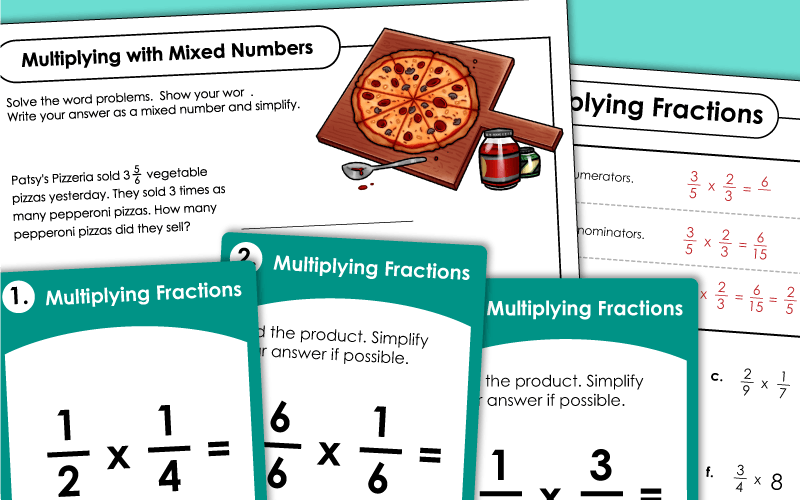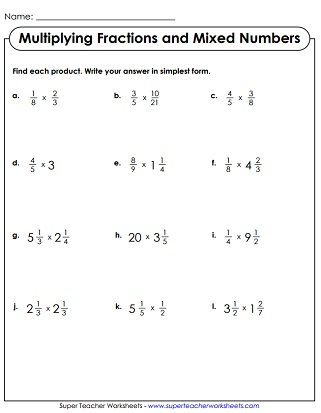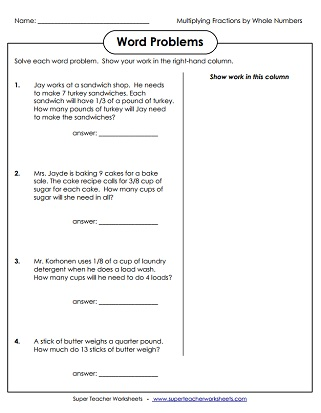# Multiplying Fractions

Students will find the products of fraction pairs in these worksheets. This page is divided into four subheaders: multiplying fractions, multiplying fractions by whole numbers, multiplying mixed numbers, and mixed skills.## Multiplying Fractions

Multiply pairs of fractions. Simplify your answers when possible.  Includes two word problems.
This worksheet has an explanation box to show students how to multiply fractions, followed by 11 practice problems.
Multiply fractions to solve each word problem. Make sure to simplify if possible, and show your work.
Cut apart and use these 30 task cards for extra practice, small group instruction, a classroom scavenger hunt, or peer study sessions.
Shapes with fractions are displayed at the top of the page. Pupils multiply fractions of similar shapes. Example: Multiply the fractions in the hexagons.

## Multiplying Fractions by Whole Numbers

This worksheet has visual representations of fraction multiplication problems.
Solve these fraction multiplication problems about a trip to the craft store.
Each of these word problems require students to multiply a whole number by a fraction or by another whole number.
These word problems require students to multiply fractions by whole numbers.
Here are 30 task cards that you can use on your document camera, for small group instruction, or for classroom games.

## Mixed Skills: Multiplying Fractions, Whole Numbers, and Mixed Numbers

Determine what happens when you multiply an improper fraction by a whole number. Will the product be greater than or less that 1? What happens when you multiply a regular fraction by a whole number? Will the product be greater or less than 1?

## Multiplying Mixed Numbers

This page shows students how to multiply mixed numbers and has 9 practice problems.
This worksheet has 12 practice problems for multiplying mixed numbers.
This card set has 30 mixed number multiplication problems. Use these cards for classroom scavenger hunts, small group instruction, peer help sessions, or morning math challenges.

Dividing Fractions

Practice dividing fractions and mixed numbers with these printable pages. Many worksheets include illustrations and models, as well as word problems.

More Fraction Worksheets

Worksheets for teaching basic fraction recognition skills and fraction concepts, as well as operations with fractions.

Reciprocal Fractions

You may also want to check out these worksheets on reciprocal fractions.

## Worksheet ImagesMy Account
Site Information